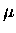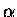Next: Delayed electron lifetime Up: Analysis I - Absolute Previous: Absolute amplitude method

# Absolute amplitude method

In this section, we shall discuss another method for the determination of the stopping fraction, referred to earlier in this chapter as the absolute amplitude method.

In this method, the same fit is performed to the decay electron time spectra as in Fig. 6.5, but we use only the amplitude information for the hydrogen component, and do not rely on the gold component in the fit. With the knowledge of the absolute electron detection efficiency, the number of muon stops in H2 can be directly measured.

The absolute efficiencies for electron detection are determined by taking advantage of a condition called delayed electron coincidence, or Del-e, and delayed telescope coincidence, Del-t (together, generally called Del cuts).  These cuts were implemented originally to suppress the muon-capture related background, particularly useful for detection of low energy fusion neutrons from dd fusion . In the Del cut, it is demanded that the electron be observed in the scintillator (telescope) within a certain time window after a hit in a detector of interest (e.g., neutron or Si). This ensures with a high probability that the event in the detector comes from aCF related process, as opposed to the capture process, since in capture the muon would be converted into a neutrino, and would not decay into an electron.

The suppression of background by more than two orders of magnitude in neutron detection was essential in order to overcome the poor signal-to-noise ratio in the dd fusion neutron measurements, but in the Si detectors, dt-fusionparticle signals can be identified without Del-e (Del-t) cuts, thanks to the good resolution of the detector. This leaves us with the ability to measure with precision the efficiency of Del-e (Del-t) cuts, from which the absolute efficiency of the electron scintillators (telescopes) can be determined.

The delayed electron (telescope) coincidence cut efficiencyis a product of the electron scintillator or telescope detection efficiency (including the solid angle), the branching ratio for electron emission , and the time cut efficiency (assuming the Huff factor is 1 for hydrogen),

 = (101) = (102)

where the intergral is over the time difference between the Si event time (tSi) and the delayed electron time (tdel), and the total electron disappearance rate is the sum of the free decay rate and muon loss rate accounting for possible muon loss, as observed in the electron lifetime. The branching ratio is defined as

 (103)

It should be noted that in our earlier paper , an incorrect expression for the delayed electron efficiencies (Eq. (4)) was used without the factor . That would be correct only when there is no muon loss (i.e. ), which is generally not the case either in Ref.  or in this thesis. For Ref. , however,was used only for relatively minor corrections related to background subtraction, hence the conclusion there should not be significantly affected. For our case, on the other hand, enters directly into the stopping fraction, as we shall see, so this distinction is rather important.

The Del cut efficiencies,, were determined experimentally from the ratio of the yield for fusion, to that for thewith a Del-e condition demanded, . The electron disappearance rate was obtained by fitting the time spectrum of the electrons detected after the fusionsignal in the Si detectors.

Thus, the electron detection efficiency can be derived via:

 = = (104)

The fit of decay electrons to exponential functions, discussed in the previous section, had given us the amplitude A2 corresponding to the hydrogen component (see Table 6.6). With A2 normalized to the number of incident muons (GMU), in was the case in Table 6.6, we have GMU, where N20 is the number of muons stopping in the hydrogen. Hence, the muon stopping fraction in hydrogen SHABS ( ), using the knowledge of the absolute efficiency of electron detectionobtained from the Del analysis, can be given by:

 SHABS = = (105)

We shall discuss each factor in Eq. 6.26 in the following subsections.Next: Delayed electron lifetime Up: Analysis I - Absolute Previous: Absolute amplitude method